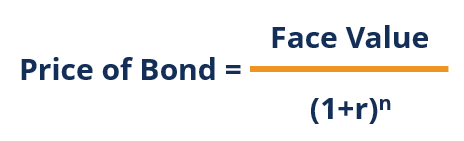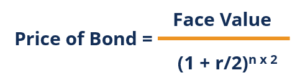# Zero-Coupon Bond

A bond that pays no interest and trades at a discount to its face value

## What is a Zero-Coupon Bond?

A zero-coupon bond is a bond that pays no interest and trades at a discount to its face value. It is also called a pure discount bond or deep discount bond. U.S. Treasury bills are an example of a zero-coupon bond.### Quick Summary:

• A zero-coupon bond is a bond that pays no interest.
• The bond trades at a discount to its face value.
• Reinvestment risk is not relevant for zero-coupon bonds, but interest rate risk is relevant for the bonds.

### Understanding Zero-Coupon Bonds

As a zero-coupon bond does not pay periodic coupons, the bond trades at a discount to its face value. To understand why, consider the time value of money.

The time value of money is a concept that illustrates that money is worth more now than an identical sum in the future – an investor would prefer to receive \$100 today than \$100 in one year. By receiving \$100 today, the investor is able to put that money into a savings account and earn interest (thereby having more than \$100 in a year’s time).

Extending the idea above into zero-coupon bonds – an investor who purchases the bond today must be compensated with a higher future value. Therefore, a zero-coupon bond must trade at a discount because the issuer must offer a return to the investor for purchasing the bond.

### Pricing Zero-Coupon Bonds

To calculate the price of a zero-coupon bond, use the following formula:Where:

• Face value is the future value (maturity value) of the bond;
• r is the required rate of return or interest rate; and
• n is the number of years until maturity.

Note that the formula above assumes that the interest rate is compounded annually. In reality, zero-coupon bonds are generally compounded semi-annually. In such a case, refer to the following formula:Note that the formula above looks similar to the previous one, with the only difference being the required rate of return (r) being divided by 2 and the number of years until maturity (n) being multiplied by two. Since the bond compounds semi-annually, we must divide the required rate of return by two and multiply the number of years until maturity by two to account for the total number of periods the bond will be compounded for.

### Example of a Zero-Coupon Bonds

#### Example 1: Annual Compounding

John is looking to purchase a zero-coupon bond with a face value of \$1,000 and 5 years to maturity. The interest rate on the bond is 5% compounded annually. What price will John pay for the bond today?

Price of bond = \$1,000 / (1+0.05)5 = \$783.53

The price that John will pay for the bond today is \$783.53.

#### Example 2: Semi-annual Compounding

John is looking to purchase a zero-coupon bond with a face value of \$1,000 and 5 years to maturity. The interest rate on the bond is 5% compounded semi-annually. What price will John pay for the bond today?

Price of bond = \$1,000 / (1+0.05/2)5*2 = \$781.20

The price that John will pay for the bond today is \$781.20.

### Reinvestment Risk and Interest Rate Risk

Reinvestment risk is the risk that an investor will be unable to reinvest a bond’s cash flows (coupon payments) at a rate equal to the investment’s required rate of return. Zero-coupon bonds are the only type of fixed-income investments that are not subject to investment risk – they do not involve periodic coupon payments.

Interest rate risk is the risk that an investor’s bond will decline in value due to fluctuations in the interest rate. Interest rate risk is relevant when an investor decides to sell a bond before maturity and affects all types of fixed-income investments.

For example, recall that John paid \$783.53 for a zero-coupon bond with a face value of \$1,000, 5 years to maturity, and a 5% interest rate compounded annually. Assume that immediately after John purchased the bond, interest rates change from 5% to 10%. In such a scenario, what would be the price of the bond?

Price of bond = \$1,000 / (1+0.10)5 = \$620.92

If John were to sell the bond immediately after purchasing it, he would realize a loss of \$162.61 (\$783.53 – \$620.92).

To conclude:

• Reinvestment risk is not relevant for zero-coupon bonds; and
• Interest rate risk is relevant for zero-coupon bonds.

### More Resources

CFI is the official provider of the Financial Modeling and Valuation Analyst (FMVA)™ certification program, designed to transform anyone into a world-class financial analyst.

To keep learning and developing your knowledge of financial analysis, we highly recommend the additional resources below:

• Continuously Compounded Return
• Effective Annual Interest Rate
• Interest Rate Risk
• 10-Year US Treasury Note

### Corporate Finance Training

Advance your career in investment banking, private equity, FP&A, treasury, corporate development and other areas of corporate finance.

Enroll in CFI’s Finance Courses to take your career to the next level! Learn step-by-step from professional Wall Street instructors today.